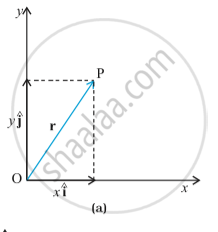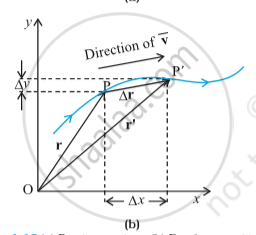ISC (Commerce) Class 11CISCE
Share

# Motion in a Plane

#### description

• Position Vector and Displacement

## Motion in a Plane

Motion in a plane is also referred to as a motion in two dimensions. For example, circular motion, projectile motion, etc. For the analysis of such type of motion, the reference point will be made of an origin and the two coordinate axes X and Y.

Motion in a plane refers to the point where we consider motion in two dimensions as only two dimensions makes a plane. Here, considering the above, we take two axes into consideration – generally X-axis or Y – axes. In an attempt to derive the equation of the motion in a plane, we must know about motion in one direction.

Position Vector and Displacement:-
The position vector r of a particle P located in a plane with reference to the origin of an x-y reference frame (Fig. below) is given by r=x hat i + y hat j
where x and y are components of r along x-, and y- axes or simply they are the coordinates of the object.(a) Position vector r.(b) Displacement Δr and average velocity v of a particle.

Suppose a particle moves along the curve shown by the thick line and is at P at time t and P′ at time t′ [Fig. (b) above]. Then, the displacement is Δr = r^' - r
and is directed from P to P′ . We can write the above equation in a component form:
Δr=(x^' hat i + y^' hat j)-(x hat i + y hat j)= hat i Δx + hat j Δ y
where Δx=x^'-x, Δy=y^'-y

### Shaalaa.com

Motion in plane part 17 (Example) [00:10:13]
S
1
0%

S